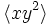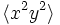# Linear representation theory of nontrivial semidirect product of Z4 and Z4

## Contents

This article gives specific information, namely, linear representation theory, about a particular group, namely: nontrivial semidirect product of Z4 and Z4.
View linear representation theory of particular groups | View other specific information about nontrivial semidirect product of Z4 and Z4

This article discusses the linear representation theory of the group nontrivial semidirect product of Z4 and Z4 (GAP ID: (16,4)), given by the presentation:$\langle x,y \mid x^4 = y^4 = e, yxy^{-1} = x^3 \rangle$

## Summary

Item Value
degrees of irreducible representations over a splitting field (such as$\overline{\mathbb{Q}}$ or$\mathbb{C}$) 1,1,1,1,1,1,1,1,2,2 (1 occurs 8 times, 2 occurs 2 times)
maximum: 2, lcm: 2, number: 10, sum of squares: 16
Schur index values of irreducible representations 1,1,1,1,1,1,1,1,1,2 (1 occurs 9 times, 2 occurs 1 time)
smallest ring of realization (characteristic zero)$\mathbb{Z}[i] = \mathbb{Z}[\sqrt{-1}] = \mathbb{Z}[t]/(t^2 + 1)$ -- ring of Gaussian integers
same as ring generated by character values
minimal splitting field, i.e., smallest field of realization (characteristic zero)$\mathbb{Q}(i) = \mathbb{Q}(\sqrt{-1}) = \mathbb{Q}[t]/(t^2 + 1)$
Same as field generated by character values, even though there is a representation of Schur index greater than one, because there are other representations whose character values anyway require us to adjoin the roots of unity that would realize this representation.
condition for a field to be a splitting field The characteristic should not be equal to 2, and the polynomial$t^2 + 1$ should split.
For a finite field of size$q$, this is equivalent to saying that$q \equiv 1 \pmod 4$
minimal splitting field in characteristic$p \ne 0,2$ Case$p \equiv 1 \pmod 4$: prime field$\mathbb{F}_p$
Case$p \equiv 3 \pmod 4$: Field$\mathbb{F}_{p^2}$, quadratic extension of prime field
smallest size splitting field field:F5, i.e., the field of five elements
degrees of irreducible representations over the rational numbers 1,1,1,1,2,2,2,4 (1 occurs 4 times, 2 occurs 3 times, 4 occurs 1 time)
number: 8
orbits of irreducible representations over a splitting field under action of automorphism group 2 orbits of size 1 of degree 1 representations, 1 orbit of size 2 of degree 1 representations, 1 orbit of size 4 of degree 1 representations, 2 orbits of size 1 of degree 2 representations
number: 6

## Representations

### Summary information

Below is summary information on irreducible representations that are absolutely irreducible, i.e., they remain irreducible in any bigger field, and in particular are irreducible in a splitting field. We assume that the characteristic of the field is not 2, except in the last column, where we consider what happens in characteristic 2.

Name of representation type Number of representations of this type Degree Schur index Criterion for field Kernel Quotient by kernel (on which it descends to a faithful representation) Characteristic 2?
trivial 1 1 1 any whole group trivial group works
sign, kernel$\langle x, y^2 \rangle$ 1 1 1 any cyclic group:Z2 works, same as trivial
sign, kernel$\langle x^2, y \rangle$ or$\langle x^2, xy \rangle$ 2 1 1 any cyclic group:Z2 works, same as trivial
kernel$\langle x \rangle$ or$\langle xy^2 \rangle$ 4 1 1 must contain a primitive fourth root of unity, i.e., the polynomial$t^2 + 1$ must split cyclic group:Z4 works, same as trivial
kernel$\langle y^2 \rangle$, obtained by composing quotient map with faithful irreducible representation of dihedral group:D8 1 2 1 any subgroup generated by a non-commutator square in nontrivial semidirect product of Z4 and Z4 dihedral group:D8 indecomposable but not irreducible
kernel$\langle x^2y^2 \rangle$, obtained by composing quotient map with faithful irreducible representation of quaternion group 1 2 2 -1 should be expressible as a sum of two squares (sufficient condition). Any finite field works central subgroup generated by a non-square in nontrivial semidirect product of Z4 and Z4 quaternion group indecomposable but not irreducible